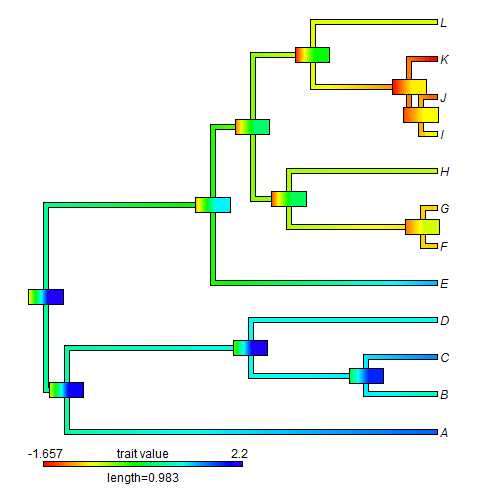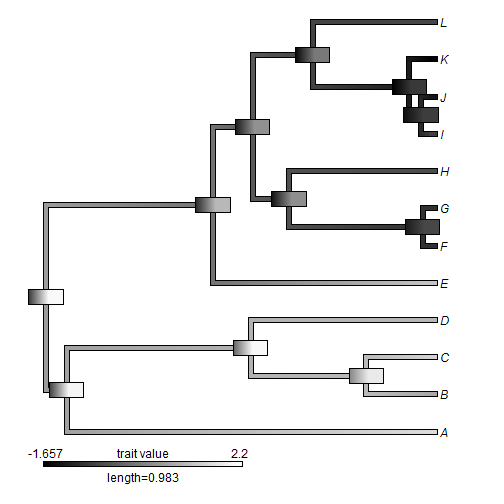## Monday, February 27, 2017

### Adding colorful error bars to internal node values using `phytools::contMap`

Today a R-sig-phylo member posted the following question:

“I was wondering about plotting the output of an ancestral state 'reconstruction' of a continuous trait while incorporating at least some of the uncertainty around the estimates.

One approach I thought of was to map the ASR onto a tree in a standard way, then at each node have essentially a mini-legend that is of a length reflecting the width of the confidence interval of the estimate at that node, and is coloured on the same colour-scale as the overall tree legend. For instance, if the colour scheme for the tree goes from blue through yellow to red as the value increases, then a node with a relatively precise and high estimate will have a short bar only ranging through different shades of red, whereas a highly uncertain low estimate will have a wider bar coloured from (say) dark blue to orange/light red. I hope that description makes sense.”

This is cannot be done automatically in phytools, but it is relatively straightforward to accomplish. For the record, I thought this would look terrible - but it actually looks better than I expected.

One key trick is that if we want our 'error bars' to show the 95% CIs for our ancestral values, we have to first find the total range of all such intervals and then use those to specify the range (argument `lims`) in our `contMap` calculation:

``````## load our package
library(phytools)
## here's our tree & data
tree
``````
``````##
## Phylogenetic tree with 12 tips and 11 internal nodes.
##
## Tip labels:
##  A, B, C, D, E, F, ...
##
## Rooted; includes branch lengths.
``````
``````x
``````
``````##          A          B          C          D          E          F
##  1.6716660  0.8798575  1.5227959  1.1046503  1.3339632 -0.9191693
##          G          H          I          J          K          L
## -0.9052098 -0.4977580 -0.6099311 -1.3487360 -1.6726186 -0.5795939
``````
``````## get ancestral states with CIs
aa<-fastAnc(tree,x,CI=TRUE)
xlim<-range(aa\$CI95) ## our range for the "contMap" object
## compute our "contMap" object
obj<-contMap(tree,x,lims=xlim,plot=FALSE)
obj
``````
``````## Object of class "contMap" containing:
##
## (1) A phylogenetic tree with 12 tips and 11 internal nodes.
##
## (2) A mapped continuous trait on the range (-1.657191, 2.200442).
``````
``````plot(obj,xlim=c(-0.12,2.2)) ## to accommodate the root node label
d<-diff(obj\$lims)
n<-length(obj\$cols)-1
lastPP<-get("last_plot.phylo",envir=.PlotPhyloEnv)
h<-max(nodeHeights(tree))
for(i in 1:tree\$Nnode){
ii<-round((aa\$CI95[i,1]-obj\$lims)/d*n)
jj<-round((aa\$CI95[i,2]-obj\$lims)/d*(n+1))
cols<-obj\$cols[ii:jj]
x=lastPP\$xx[i+Ntip(tree)]-0.025*h,
y=lastPP\$yy[i+Ntip(tree)],title="",subtitle="",lims=NULL,
lwd=14)
}
``````That's it. If we want to change our color scheme, that is also no problem:

``````obj<-setMap(obj,colors=c("black","white"))
plot(obj,xlim=c(-0.12,2.2))
d<-diff(obj\$lims)
n<-length(obj\$cols)-1
lastPP<-get("last_plot.phylo",envir=.PlotPhyloEnv)
h<-max(nodeHeights(tree))
for(i in 1:tree\$Nnode){
ii<-round((aa\$CI95[i,1]-obj\$lims)/d*n)
jj<-round((aa\$CI95[i,2]-obj\$lims)/d*(n+1))
cols<-obj\$cols[ii:jj]
x=lastPP\$xx[i+Ntip(tree)]-0.025*h,
y=lastPP\$yy[i+Ntip(tree)],title="",subtitle="",lims=NULL,
lwd=14)
}
``````Neat.

Obviously, any of the parameters of the error bars - their length (here set to 5% of the total tree length), width, etc., can easily be changed by modifying the code above.

The tree & data for this exercise were simulated as follows:

``````tree<-pbtree(n=12,tip.label=LETTERS[1:12])
x<-fastBM(tree)
``````

That's all.